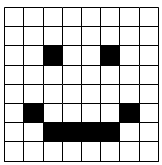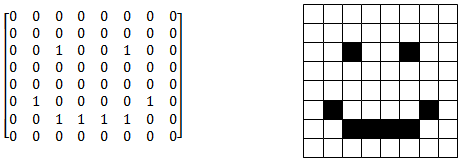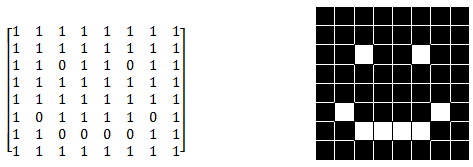# 8.3 Matrices to Represent Data

$$\newcommand{\vecs}{\overset { \rightharpoonup} {\mathbf{#1}} }$$ $$\newcommand{\vecd}{\overset{-\!-\!\rightharpoonup}{\vphantom{a}\smash {#1}}}$$$$\newcommand{\id}{\mathrm{id}}$$ $$\newcommand{\Span}{\mathrm{span}}$$ $$\newcommand{\kernel}{\mathrm{null}\,}$$ $$\newcommand{\range}{\mathrm{range}\,}$$ $$\newcommand{\RealPart}{\mathrm{Re}}$$ $$\newcommand{\ImaginaryPart}{\mathrm{Im}}$$ $$\newcommand{\Argument}{\mathrm{Arg}}$$ $$\newcommand{\norm}{\| #1 \|}$$ $$\newcommand{\inner}{\langle #1, #2 \rangle}$$ $$\newcommand{\Span}{\mathrm{span}}$$ $$\newcommand{\id}{\mathrm{id}}$$ $$\newcommand{\Span}{\mathrm{span}}$$ $$\newcommand{\kernel}{\mathrm{null}\,}$$ $$\newcommand{\range}{\mathrm{range}\,}$$ $$\newcommand{\RealPart}{\mathrm{Re}}$$ $$\newcommand{\ImaginaryPart}{\mathrm{Im}}$$ $$\newcommand{\Argument}{\mathrm{Arg}}$$ $$\newcommand{\norm}{\| #1 \|}$$ $$\newcommand{\inner}{\langle #1, #2 \rangle}$$ $$\newcommand{\Span}{\mathrm{span}}$$$$\newcommand{\AA}{\unicode[.8,0]{x212B}}$$

A matrix is a rectangular array of numbers representing data in a variety of forms. Computers work very heavily with matrices because operations with matrices are efficient with memory. Matrices can represent statistical data with numbers, but also graphical data with pictures.

How might you use a matrix to write the following image as something a computer could recognize and work with?## Introduction to Matrices

A matrix is a means of storing information effectively and efficiently. The rows and columns each mean something very specific and the location of a number is just as important as its value. The following are all examples of matrices:

$$\left[\begin{array}{lll} 1 & 2 & 3 \\ 4 & 5 & 6 \end{array}\right]$$, $$\left[\begin{array}{lll} 1 & 0 & 0 \\ 0 & 1 & 0 \\ 0 & 0 & 1 \end{array}\right]$$, $$\left[\begin{array}{cccc} 2 & 3 & 4 & 5 \\ 0 & 4 & 5 & 0 \\ 0 & 0 & 2 & -9 \\ 0 & 0 & 0 & 10 \end{array}\right]$$

The entries in a matrix can be written out using brackets like [ ], but they can also be described individually using a set of 2 subscript indices $$i$$ and $$j$$ that stand for the row number and the column number. Alternatively, the matrix can be named with just a capital letter like $$A$$.

A=$$\left[a_{i j}\right]=\left[\begin{array}{ll} a_{11} & a_{12} \\ a_{21} & a_{22} \end{array}\right]$$

Square matrices have the same number of rows as columns. The order of a matrix describes the number of rows and the number of columns in the matrix. The following matrix is said to have order $$2 \times 3$$ because it has two rows and three columns. A $$1 \times 1$$ matrix is just a regular number.

$$\left[\begin{array}{lll} 1 & 2 & 3 \\ 4 & 5 & 6 \end{array}\right]$$

A symmetric matrix is a special type of square matrix that has reflection symmetry across the main diagonal. The identity matrix is an example of a symmetric matrix.

The identity matrix of order $$n \times n$$ has zeros everywhere except along the main diagonal where it has ones. Just like the number 1 has an important property with numbers, the identity matrix of any order has special properties as well.

,$$\left[\begin{array}{ll} 1 & 0 \\ 0 & 1 \end{array}\right]$$,$$\left[\begin{array}{lll} 1 & 0 & 0 \\ 0 & 1 & 0 \\ 0 & 0 & 1 \end{array}\right]$$

When you turn the rows of a matrix into the columns of a new matrix, the two matrices are

transpositions of one another. The superscript $$T$$ stands for transpose. Sometimes using the

transpose of a matrix is more useful than using the matrix itself.

$$\begin{array}{l} A=\left[\begin{array}{lll} 1 & 2 & 3 \\ 4 & 5 & 6 \end{array}\right] \\ A^{T}=\left[\begin{array}{ll} 1 & 4 \\ 2 & 5 \\ 3 & 6 \end{array}\right] \end{array}$$

A triangular matrix is not a matrix in the shape of a triangle. Rather, a lower triangular matrix is a square matrix where every entry below the diagonal is zero. An upper triangular matrix is a square matrix where every entry above the diagonal is zero. The following is a lower triangular matrix. When you work with solving matrices, look for triangular matrices because they are much easier to solve.

$$\left[\begin{array}{cccc} 2 & 3 & 4 & 5 \\ 0 & 4 & 5 & 0 \\ 0 & 0 & 2 & -9 \\ 0 & 0 & 0 & 10 \end{array}\right]$$

A diagonal matrix is both upper and lower triangular which means all the entries except those along the diagonal are zero. The identity matrix is a special case of a diagonal matrix.

## Examples

##### Example 1

Earlier, you were asked how you might use a matrix to write the following image as something a computer could recognize and work with.By writing every hollow square as a 0 and a blank square as a 1 a computer could read the picture:

When you use computers to manipulate images, the computer just manipulates the numbers. In this case, if you swap zeros and ones, you get the negative image.Real photos and computer images have matrices that are much larger and include more numbers than just zero and one to account for more colors.

##### Example 2

Kate runs three bakeries and each bakery sells bagels and muffins. The rows represent the bakeries and the columns represent bagels (left) and muffins (right) sold. Answer the following questions about Kate’s sales.

K=$$\left[\begin{array}{cc} 144 & 192 \\ 115 & 127 \\ 27 & 34\end{array}\right]$$

1. What does 127 represent?

a. 127 represents the number of muffins that Kate sold in her second location. You know this because it is in the muffin column and the second row.

2. How many muffins did Kate sell in total?

a. The total muffins sold is equal to the sum of the right hand column. $$192+127+34=353$$

3. How many bagels did Kate sell in her first location?

a. Kate sold 144 bagels at her first location.

4. Which location is doing poorly?

a. The third location is doing much worse than the other two locations.

##### Example 3

Identify the order of the following matrices

$$A=\left[\begin{array}{llll}1 & 3 & 4 & 7\end{array}\right], \quad B=\left[\begin{array}{ccc}21 & 45 & 1 \\ 34 & 1 & 5\end{array}\right], \quad C=\left[\begin{array}{cc}25 & 235 \\ 562 & 562 \\ 4 & 413 \\ 454 & 33 \\ 1 & 141\end{array}\right]$$

$$A$$ is $$1 \times 4, B$$ is $$2 \times 3, C$$ is $$5 \times 2$$. Note that $$4 \times 1,3 \times 2,2 \times 5$$ are not the same orders and would be incorrect.

##### Example 4

Write out the $$5 \times 4$$ matrix whose entries are $$a_{i j}=\frac{i+j}{j}$$

$$\left[\begin{array}{ccccc} 2 & \frac{3}{2} & \frac{4}{3} & \frac{5}{4} & \frac{6}{5} \\ 3 & 2 & \frac{5}{3} & \frac{3}{2} & \frac{7}{5} \\ 4 & \frac{5}{2} & 2 & \frac{7}{4} & \frac{8}{5} \\ 5 & 3 & \frac{7}{3} & 2 & frac{9}{5} \end{array}\right]$$

##### Example 5

Create a $$3 \times 3$$ matrix for each of the following:

a. Diagonal Matrix

$$\left[\begin{array}{lll}4 & 0 & 0 \\ 0 & 3 & 0 \\ 0 & 0 & 5\end{array}\right]$$

b. Lower Triangular

$$\left[\begin{array}{ccc}4 & 1 & 1 \\ 0 & 3 & 14 \\ 0 & 0 & 5\end{array}\right]$$

c. Symmetric

$$\left[\begin{array}{ccc}4 & 1 & 1 \\ 1 & 3 & 14 \\ 1 & 14 & 5\end{array}\right]$$

d. Identity:

$$\left[\begin{array}{lll}1 & 0 & 0 \\ 0 & 1 & 0 \\ 0 & 0 & 1\end{array}\right]$$

Note that while the identity matrix does technically work for all the parts of this problem, it does not highlight the differences between each definition.

##### Review

State the order of each of the following matrices:

1. $$A=\left[\begin{array}{llll}4 & 2 & 4 & 7 \\ 5 & 2 & 1 & 0\end{array}\right]$$

2. $$B=\left[\begin{array}{cc}0 & 1 \\ 34 & 1\end{array}\right]$$

3. $$C=\left[\begin{array}{cc}2 & 62 \\ 14 & 3 \\ 4 & 3 \\ 1 & 11\end{array}\right]$$

4. $$D=\left[\begin{array}{ccc}12 & 0 & 2 \\ 0 & 3 & 3 \\ 4 & 0 & 1 \\ 1 & 4 & 0\end{array}\right]$$

5. $$E=\left[\begin{array}{ll}1 & 11\end{array}\right]$$

6. Give an example of a $$1 \times 1$$ matrix.

7. Give an example of a $$3 \times 2$$ matrix.

8. If a symmetric matrix is also lower triangular, what type of matrix is it?

9. Write out the $$2 \times 3$$ matrix whose entries are $$a_{i j}=i-j$$.

Morgan worked for three weeks during the summer earning money on Mondays, Tuesdays, Wednesdays, Thursdays, and Fridays. The following matrix represents his earnings.

$$\left[\begin{array}{lll} 24 & 22 & 32 \\ 25 & 28 & 30 \\ 30 & 28 & 32 \\ 10 & 15 & 19 \\ 35 & 32 & 30 \end{array}\right]$$

10. What do the rows and columns represent?

11. How much money did Morgan make in the first week?

12. How much money did Morgan make on Tuesdays?

13. What day of the week was most profitable?

14. What day of the week was least profitable?

15. Is the following a matrix? Explain.

$$\left[\begin{array}{cc} \text { dogs } & 0 \\ \text { cats } & 3 \\ \text { sheep } & 0 \\ \text { ducks } & 4 \end{array}\right]$$

This page titled 8.3 Matrices to Represent Data is shared under a CK-12 license and was authored, remixed, and/or curated by CK-12 Foundation via source content that was edited to the style and standards of the LibreTexts platform; a detailed edit history is available upon request.